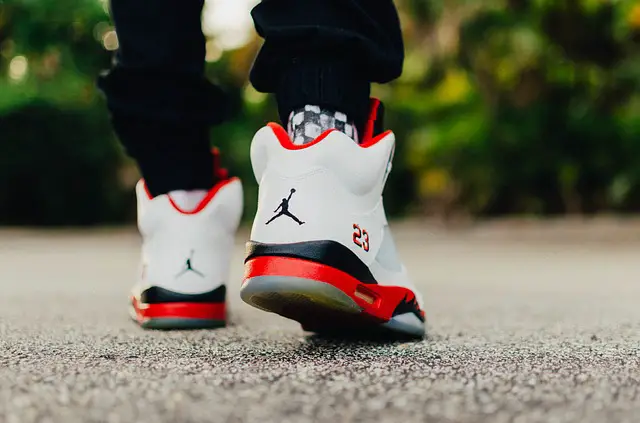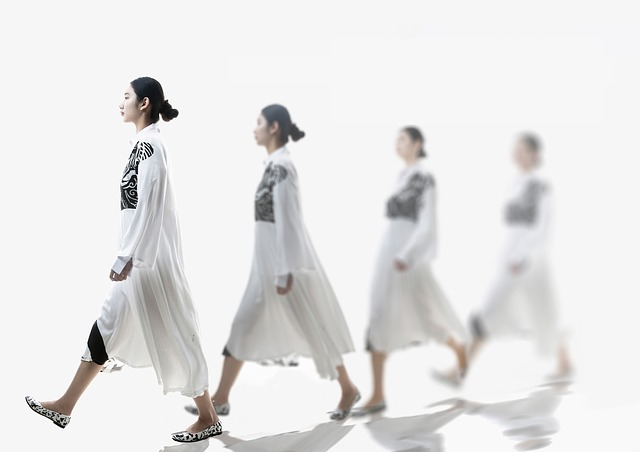# How Friction helps in walking?-explanation with free body diagram

## How friction helps in walking

“If Friction is a force that opposes motion then how friction helps in walking?” As we walk we apply a contact force on the surface below and that surface also exerts an equal and opposite contact force on our foot or shoes. Depending on the position and angle of our foot, this reaction contact force applied on our foot helps us to move forward as well as saves us from slipping and falling. This is how friction helps walking, in simple words.Above said answer can be easily understood if we take help of Free Body Diagram and Vector Resolution of contact Forces.

## How is friction useful sometimes? – Friction is a necessary evil

Very often similar questions come from the Physics students. Some asks, “How is friction useful sometimes? or “How does friction help us?”

If we go by the common notion we have about Friction, certainly these questions may arise in young and eager minds who are trying to grasp the fundamentals of physics. Well, now to start off the discussion, we can say that Friction is a necessary evil.

Many times it causes lots of damage, but there are cases where friction comes up as a savior.

In this post we’ll try to explore multiple steps involved in the walking process and draw Free Body Diagrams (FBD) for each to understand how different force components are playing in the walking process and how and where Friction helps in walking.

But before that we will have a quick revision on the definition of Friction and different types of it. So lets see how friction is a necessary evil.

## What is Friction?

Friction is a force which acts along the common surface of two objects when one object tends to move or moves actually with respect to the other. The direction of this Friction or Frictional force will be opposite to the direction of the motion.

Friction occurs because of the electrical forces between the surfaces where two objects are in contact.

Want to have a better definition: Frictional force Definition

## Types of Friction

Friction may be of different types depending on when and how it evolves and varies.

Static friction: Static friction, is the force that tends to prevent a stationary object to start moving.

The maximum static friction is called the limiting friction or starting friction. It is the amount of force that must be overcome to start a stationary object moving.
Example: attempting to move a heavy cabinet across a floor.

Kinetic Friction: Once the force applied to an object crosses the limiting friction, the
object starts moving. Then, moving or kinetic friction replaces static friction.

Kinetic friction is the force that acts against an object’s motion in a direction opposite to the direction of motion.

If the applied force has the same magnitude as the kinetic friction while moving an object horizontally, then the net force on the object will be zero and the moving object will maintain uniform velocity.

Kinetic friction may be of different types depending on the situation like Sliding friction, rolling friction and fluid friction.

## How friction helps in Walking – 2 major steps

Our walking consists of a series of different steps among which we have selected two major steps which really takes help of Friction to have a balanced stature and then move forward.

From these 2 steps it will be clear how friction helps us in walking or how friction is useful sometimes.

### Step 1-role of friction in walking

As we apply a force F on the floor (see fig 1), the ground exerts a equal and opposite force F1 as per Newton’s third law of motion.

That means, F1 = – F . Here F1 is the reaction force of applied force F.

Now using Vector Resolution of force, lets resolve the applied force F and the reaction force F1 one by one.

(a) Applied force F is resolved into 2 components. The perpendicular downward component acts as the Weight W. And the horizontal component acting backwards is the horizontal effective push given by the foot on the floor.

(b) Now lets resolve the Reaction force F1 into 2 components.

Its perpendicular component (shown as N in the figure) is the Normal reaction. The horizontal component acts as the Friction FR.

So you can see very easily that Friction (FR) just acts horizontally in the opposite direction of the effective horizontal push mentioned in the above section. This Friction (FR) supplies the forward push to move ahead while walking.

### Step 2 – how does friction help us?

In step 2 the position of the foot is different than step 1.

Its more of an effort to balance the position.

As we apply a force F on the floor (see fig 2), the ground exerts a equal and opposite force F1.

Here also, F1 = – F . Here F1 is the reaction force of applied force F.

Now using Vector Resolution of force, lets resolve the applied force F and the reaction force F1 one by one like we did in step 1.

(a) Applied force F is resolved into 2 components.

The perpendicular downward component acts as the Weight W. And the horizontal component  acting forward is the horizontal effective push given by the foot on the floor.

This horizontal push, if not counterbalanced, will make us slip and fall. But fortunately friction is there to counterbalance this. (see the section below:b)

(b) Now lets resolve the Reaction force F1 into 2 components.

Its perpendicular component (shown as N in the figure) is the Normal reaction. The horizontal component towards the reverse/backward direction here acts as the Friction FR.

So you can see here also that Friction (FR) acts horizontally in the opposite direction of the effective horizontal push.

But in this case the Friction (FR) supplies the backward balancing push to avoid slipping while walking. See here how friction is useful sometimes.

### Conclusion: Friction helps in walking

So in step 1 (fig 1)we see friction helping us to provide a forward push to move ahead while walking.

And in step 2 (fig 2) its supplying a backward balancing force which helps us to balance and not slip.

This simply shows how friction helps us in walking. This also explains that friction is useful sometimes !

Read another relevant post on friction here:

Pushing a car

Newton’s third law of motion